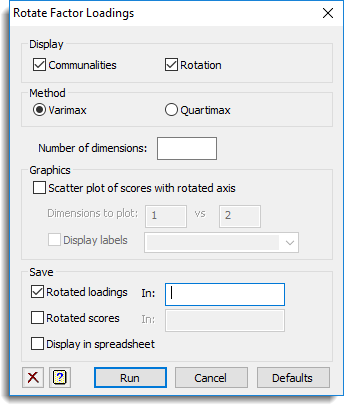1. Home

This dialog lets you rotate factor loadings from a principal components analysis according to either the Varimax or Quartimax criterion. Principal components analysis defines a set of dimensions (sometimes called axes) that are linear combinations of the original variables. The individual coefficients of these combinations are called loadings, and can be used to interpret the dimensions.## Display

This specifies which items of output are to be produced by the analysis.

 Communalities Displays the communalities of the variables Rotation Displays the rotated factors

## Method

Controls the method used for the factor rotation. The Varimax rotation, maximizes the variance of the squares of the loadings within each new dimension: the effect of this rotation should be to spread out the squared-loadings to the extremes of their range. The Quartimax rotation uses the fourth power of the loadings instead of the second power.

## Number of dimensions

This specifies the number of dimensions to rotate from the original loadings (the other dimensions are left unchanged).

## Graphics

Specifies graphical display of the results from the analysis.

 Scatter plot of scores with rotated axis Draws a scatter plot matrix of the principal component scores with the rotated axis. The axis dimensions to be displayed in the plot can be specified using the Dimensions to plot option Dimensions to plot Controls the axis dimensions to be used in the plot. The axis dimension should be less than or equal to the number specified in the Number of dimensions field in the principal components options Display labels Specify a text containing labels for the individual points displayed in the plots. The text should be equal in length to the data variates

## Save

This lets you save results from the factor rotation in Genstat data structures. After selecting the appropriate boxes, you need to type the names for the identifiers of the data structures into the corresponding In: fields.